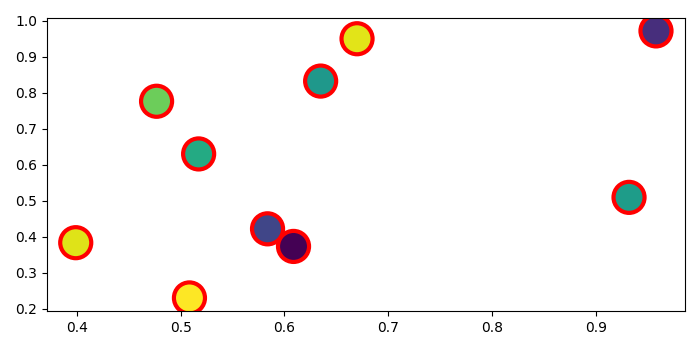# How to set the border color of the dots in matplotlib's scatterplots?

To set the border color of the dots in matplotlib scatterplots, we can take the following steps −

• Set the figure size and adjust the padding between and around the subplots.
• Initialize a variable "N" to store the number of sample data.
• Create x and y data points using numpy.
• Plot the x and y data points using scatter() method. To set the border color of the dots, use the edgecolors parameter in the scatter() method. Here, we have used "red" as the border color of the dots by using edgecolors='red'.
• To display the figure, use show() method.

## Example

import numpy as np
from matplotlib import pyplot as plt

plt.rcParams["figure.figsize"] = [7.00, 3.50]
plt.rcParams["figure.autolayout"] = True

N = 10

x = np.random.rand(N)
y = np.random.rand(N)

plt.scatter(x, y, s=500, c=np.random.rand(N),
edgecolors='red', linewidth=3)

plt.show()

## Output

It will produce the following output